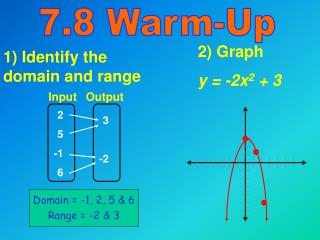DownloadDownload Presentation1) Identify the domain and range

# 1) Identify the domain and range

Download Presentation## 1) Identify the domain and range

- - - - - - - - - - - - - - - - - - - - - - - - - - - E N D - - - - - - - - - - - - - - - - - - - - - - - - - - -
##### Presentation Transcript

1. Input Output 2 5 -1 6 3 -2 Domain = -1, 2, 5 & 6 Range = -2 & 3 7.8 Warm-Up 2) Graph y = -2x2 + 3 1) Identify the domain and range

2. Objective-Students will learn to graph functions of the form y = a x – h + k and y = a 3 x – h + k. y y (1,1) (1,1) (-1,-1) (0,0) x (0,0) x 7.8 Graphing Square Root and Cube Root Functions Domain: x > 0, Range: y > 0 Domain and range: all real numbers

3. Example 1 Comparing Two Graphs Describe how to create the graph of y = x + 2 – 4 from the graph of y = x . Solution h = -2 and k = -4 shift the graph to the left 2 units & down 4 units

4. To graph y = a x - h + k or y = a 3 x - h + k, follow these steps. STEP uSketch the graph of y = a x or y = a 3 x . Graphs of Radical Functions STEP vShift the graph h units horizontally and k units vertically

5. Solution • Sketch the graph of • y = -3 x (dashed). • It begins at the origin and passes through point (1,-3). (1, 3) (0,0) 2) For y = -3 x – 1 + 3, h = 1 & k = 3. Shift both points 1 to the right and 3 up. (2,0) (1,-3) vExample 2 Graphing a Square Root Graph y = -3 x – 1 + 3 .

6. (2, 1) (0,0) (3,3) (1,2) Graphing a Square Root Graph y = 2 x – 2 + 1 .

7. Solution • Sketch the graph of • y = 2 3 x (dashed). • It passed through the origin & the points • (1, 2) & (-1, -2). (1, 2) (0,0) 2) For y = 2 x + 3 – 4, h = -3 & k = -4. Shift the three points Left 3 and Down 4. (-2,-2) (-1,-2) (-3,-4) (-4,-6) v Example 3 Graphing a Cube Root Graph y = 2 3 x + 3 – 4 .

8. (1, 3) (0,0) (3,4) (-1,-3) (2,1) (1,-2) Graphing a Cube Root Graph y = 3 3 x – 2 + 1

9. 7.8 Homework Pg. 423 # 1 – 23* odd

10. 7.8 Guided Practice Pg. 423 # 3 – 6 and 18 – 21